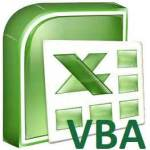# Excel VBA usedRange Property and reset usedRangeThis tutorial explains how to use Excel VBA usedRange Property to find the last used row and column number, and reset usedRange. You may also want to read: Excel VBA custom Function last row and last column Excel delete blank rows and blank cells before import into Access Excel VBA UsedRange Property Excel VBA UsedRange is a worksheet Property, it returns the area Range bounded by first used cell and last used cell. "Used Cell" is defined as Cell containing formula, formatting, value th...
More

# Excel VBA custom Function last row and last columnThis tutorial explains how to create a custom VBA Function to find the last row and last column number in Excel worksheet. You may also want to read: Excel find the last row using Worksheet formula Excel VBA custom Function last row and last column In Excel VBA, we always need to loop through each Cell to perform some actions. For Range that you already know, you can easily use For...Each Loop to loop through each Cell for a specified Range as below For Each rng in Range("A1:C10") ...
More